Question

# Question 7 of 22 (1 point) Attempt 1 of Unlimited View question in a pour 82...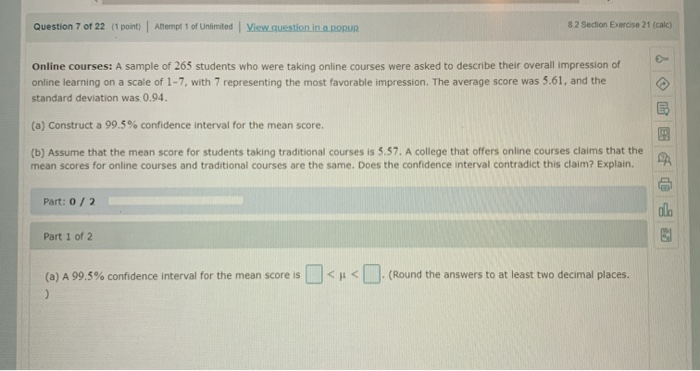Question 7 of 22 (1 point) Attempt 1 of Unlimited View question in a pour 82 Section Exercise 21 (cale) Online courses: A sample of 265 students who were taking online courses were asked to describe their overall impression of online learning on a scale of 1-7, with 7 representing the most favorable impression. The average score was 5.61, and the standard deviation was 0.94. (a) Construct a 99.5% confidence interval for the mean score. (b) Assume that the mean score for students taking traditional courses is 5.57. A college that offers online courses claims that the mean scores for online courses and traditional courses are the same. Does the confidence interval contradict this claim? Explain. Part: 0/2 olo Part 1 of 2 (a) A 99.5% confidence interval for the mean score is <<.(Round the answers to at least two decimal places.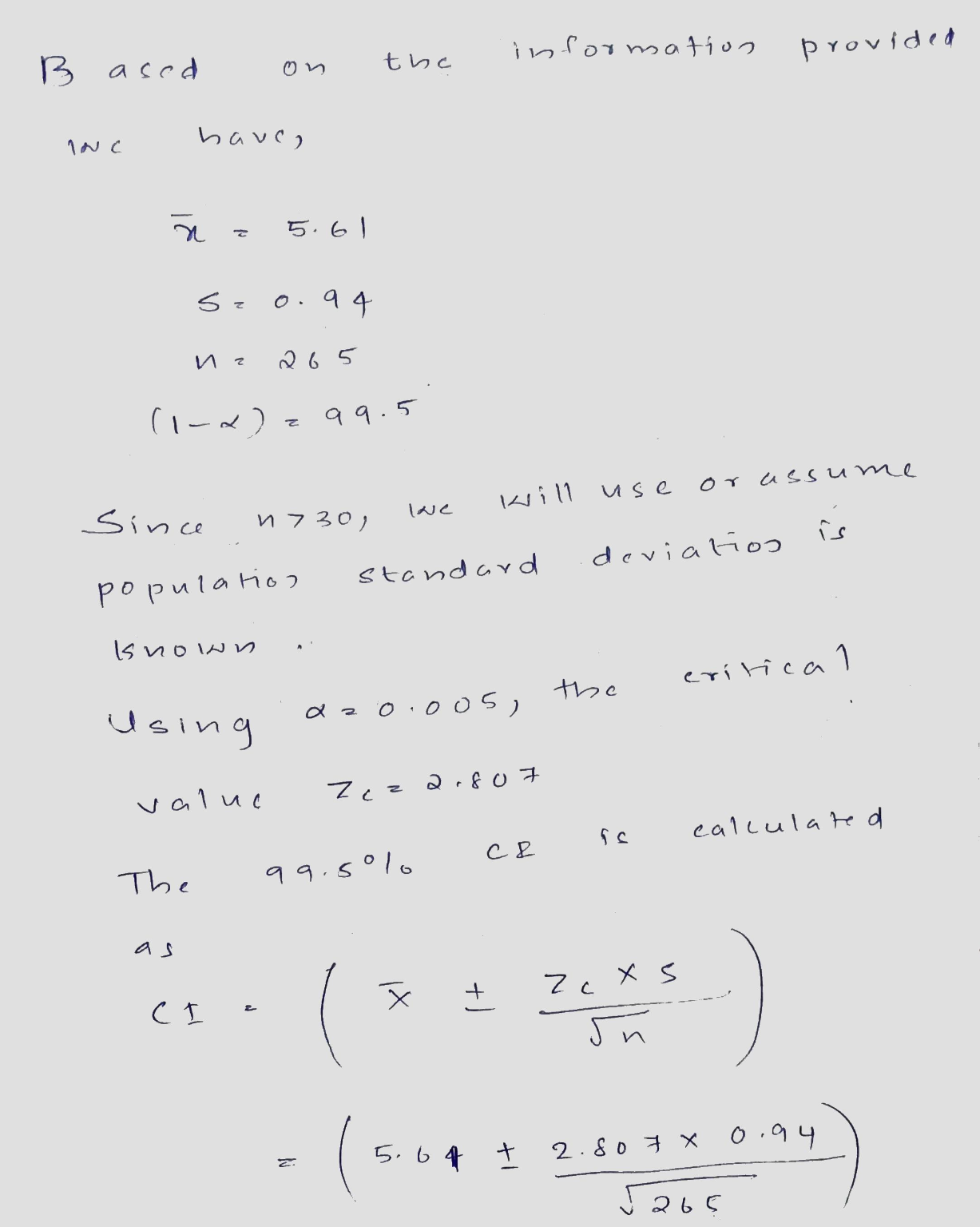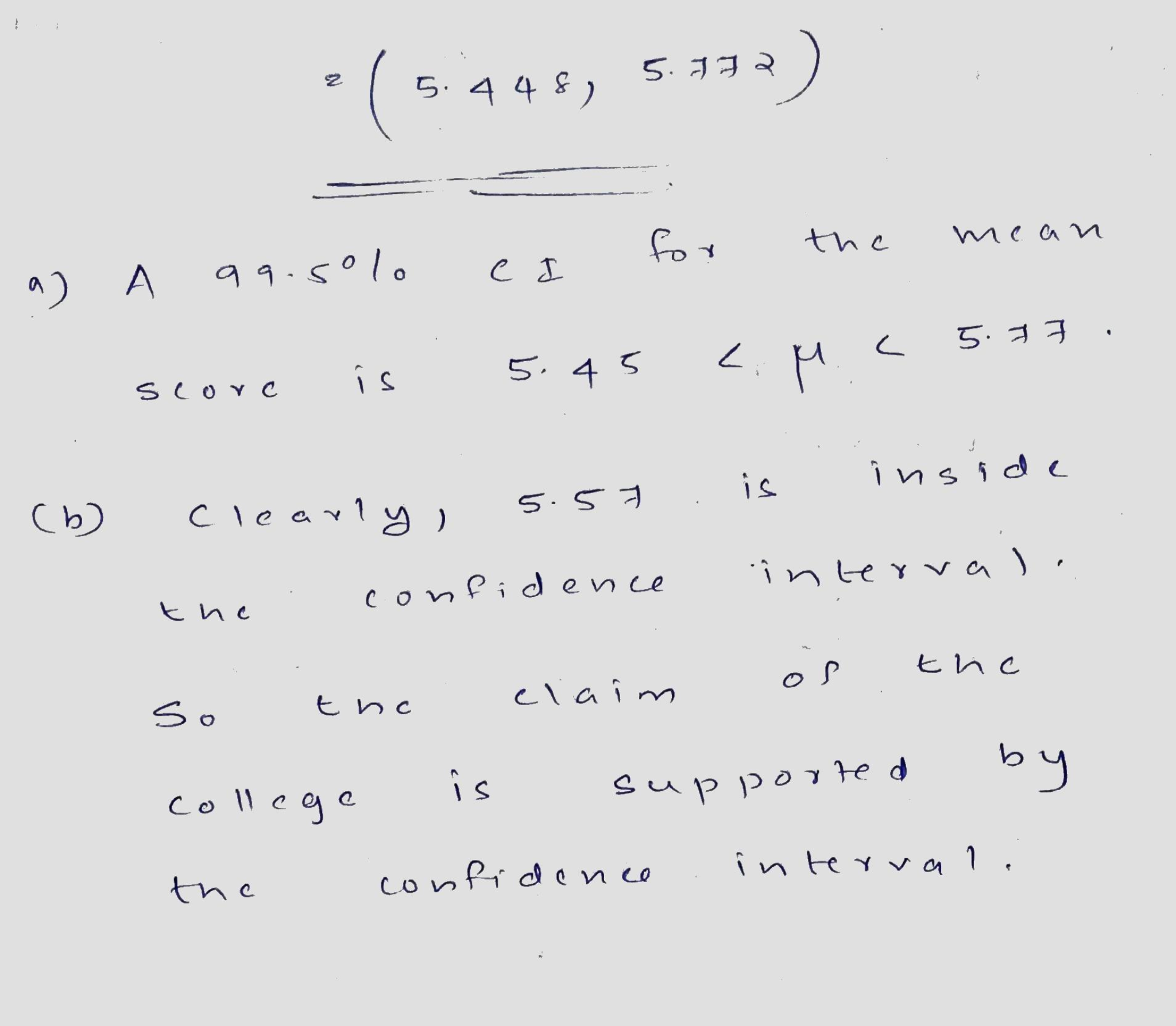#### Earn Coins

Coins can be redeemed for fabulous gifts.

Similar Homework Help Questions
• ### Question 8 of 18 (1 point) Attempt 1 of Unlimited View question in a popur! 72...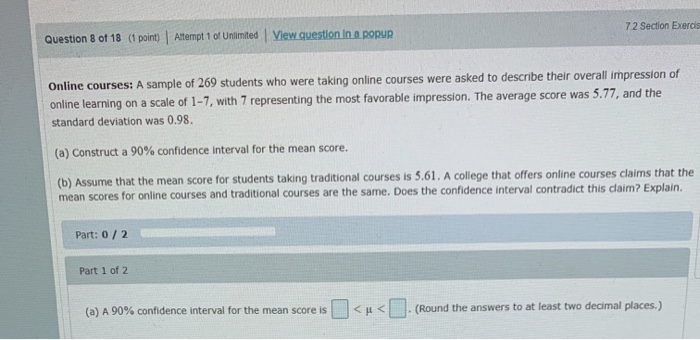Question 8 of 18 (1 point) Attempt 1 of Unlimited View question in a popur! 72 Section Exerci Online courses: A sample of 269 students who were taking online courses were asked to describe their overall impression of online learning on a scale of 1-7, with 7 representing the most favorable impression. The average score was 5.77, and the standard deviation was 0.98. (a) Construct a 90% confidence interval for the mean score. (b) Assume that the mean score for...

• ### A sample of 250 students who were taking online courses were asked to describe their overall...

A sample of 250 students who were taking online courses were asked to describe their overall impression of online learning on a scale of 1-7 with 7 representing the most favorable impression. The average score was 4.98 and the standard deviation was 0.87. Construct a 90% confidence interval for the mean score. Round your final answers to 3 decimal places. The 90% confidence interval for the mean score is: ( , )

• ### Question 1 of 13 (1 point) Attempt 1 of Unlimited View question in a popup 8.1...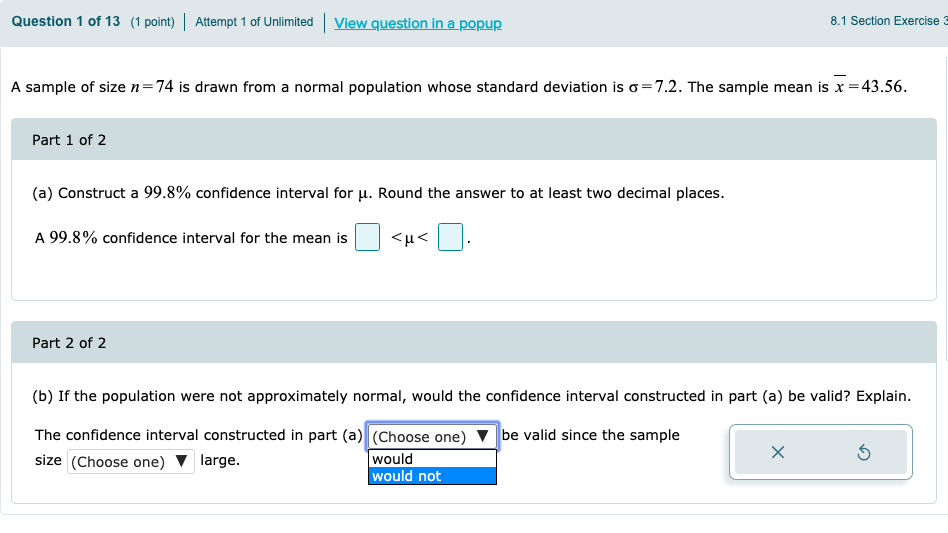Question 1 of 13 (1 point) Attempt 1 of Unlimited View question in a popup 8.1 Section Exercise A sample of size n=74 is drawn from a normal population whose standard deviation is o=7.2. The sample mean is x =43.56. Part 1 of 2 (a) Construct a 99.8% confidence interval for u. Round the answer to at least two decimal places. A 99.8% confidence interval for the mean is <u< . Part 2 of 2 (b) If the population were...

• ### Question 1 of 7 (1 point) Attempt 1 of 1 View question in a pour och...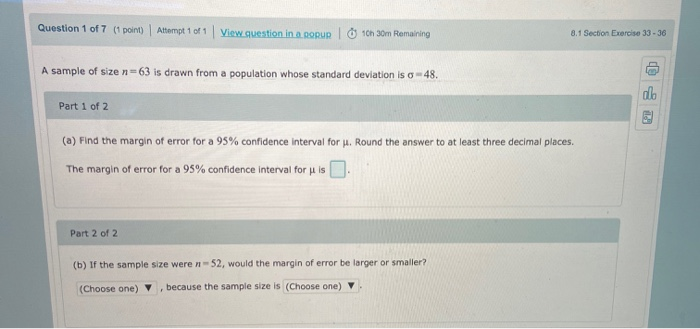Question 1 of 7 (1 point) Attempt 1 of 1 View question in a pour och 30m Remaining 8.1 Section Exercise 33-36 A sample of size n=63 is drawn from a population whose standard deviation is o - 48. co Part 1 of 2 (a) Find the margin of error for a 95% confidence interval for J. Round the answer to at least three decimal places. The margin of error for a 95% confidence interval for u is Part 2...

• ### stion 14 of 18 (1 point) Attempt 1 of Unlimited View question in a popun iart...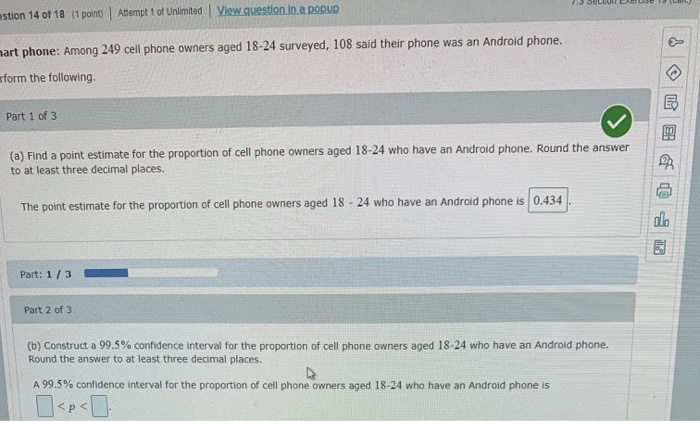stion 14 of 18 (1 point) Attempt 1 of Unlimited View question in a popun iart phone: Among 249 cell phone owners aged 18-24 surveyed, 108 said their phone was an Android phone. rform the following. Part 1 of 3 (a) Find a point estimate for the proportion of cell phone owners aged 18-24 who have an Android phone. Round the answer to at least three decimal places. The point estimate for the proportion of cell phone owners aged 18...

• ### Question 7 of 7 (1 point) Attempt 1 of Unlimited View question in a ponun 8.3...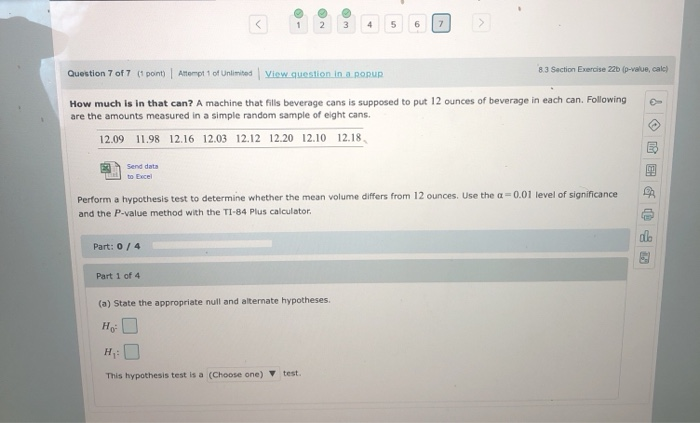Question 7 of 7 (1 point) Attempt 1 of Unlimited View question in a ponun 8.3 Section Exercise 22 p-value, cale) How much is in that can? A machine that fills beverage cans is supposed to put 12 ounces of beverage in each can. Following are the amounts measured in a simple random sample of eight cans. 12.09 11.98 12.16 12.03 12.12 12.20 12.10 12.18 Sene date Perform a hypothesis test to determine whether the mean volume differs from 12...

• ### Question 13 of 14 (1 point) Attempt 1 of Unlimited View question in a pour 8.2...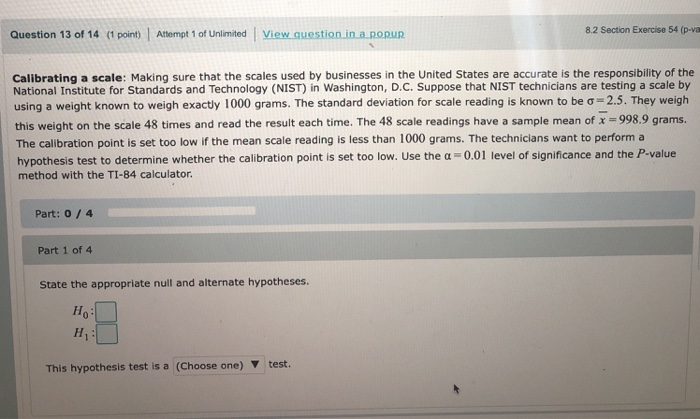Question 13 of 14 (1 point) Attempt 1 of Unlimited View question in a pour 8.2 Section Exercise 54 (p-va Calibrating a scale: Making sure that the scales used by businesses in the United States are accurate is the responsibility of the National Institute for Standards and Technology (NIST) in Washington, D.C. Suppose that NIST technicians are testing a scale by using a weight known to weigh exactly 1000 grams. The standard deviation for scale reading is known to be...

• ### Question 14 of 20 (1 point) Attempt 1 of Unlimited View question in a pour 6...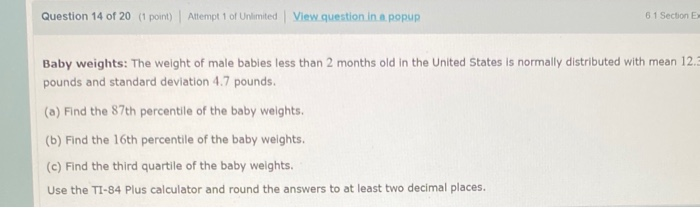Question 14 of 20 (1 point) Attempt 1 of Unlimited View question in a pour 6 1 Section E Baby weights: The weight of male babies less than 2 months old in the United States is normally distributed with mean 12.3 pounds and standard deviation 4.7 pounds. (a) Find the 87th percentile of the baby weights. (b) Find the 16th percentile of the baby weights. (c) Find the third quartile of the baby weights. Use the TI-84 Plus calculator and...

• ### Question 4 of 18 (1 point) Attempt 2 of Unlimited View question. In a popuR! 7.1 Section Exercise 37-38 A sample...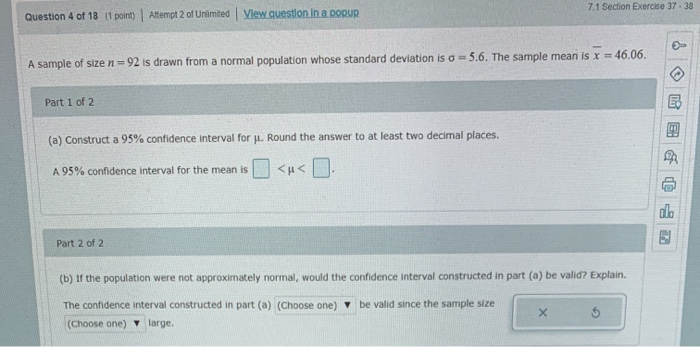Question 4 of 18 (1 point) Attempt 2 of Unlimited View question. In a popuR! 7.1 Section Exercise 37-38 A sample of size n = 92 is drawn from a normal population whose standard deviation is a = 5.6. The sample mean is x = 46.06. Part 1 of 2 (a) Construct a 95% confidence interval for Jl. Round the answer to at least two decimal places. A 95% confidence interval for the mean is <u< . Part 2 of...

• ### Question 16 of 18 (1 point) Attempt 1 of Unlimited 74 Section Exercise 12 (cal Ages...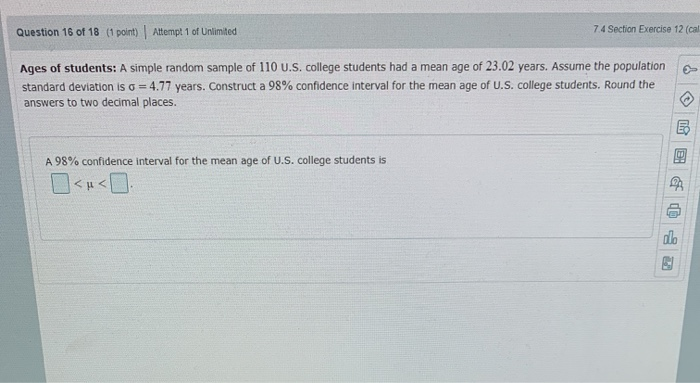Question 16 of 18 (1 point) Attempt 1 of Unlimited 74 Section Exercise 12 (cal Ages of students: A simple random sample of 110 U.S. college students had a mean age of 23.02 years. Assume the population standard deviation is o = 4.77 years. Construct a 98% confidence interval for the mean age of U.S. college students. Round the answers to two decimal places. A 98% confidence interval for the mean age of U.S. college students is